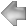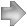## The Perfect Numbers and Pascal's Triangle

I originally wrote this paper while I was a senior in High School (1974). I submitted it to the Pi Mu Epsilon Journal where it was printed in the Spring 1978, Volume 6, Number 8 issue (page 459). This paper won the award for outstanding paper of 1978 (also known as the Richard V. Andree Award) from Pi Mu Epsilon.

Theorem: All even perfect numbers are on the third diagonal of Pascal's Triangle.

Proof: The proof is detailed in the following paper.

 The Perfect Numbers and Pascal's Triangle by Robert A. Antol Iowa State University original copyright (c) 1974

The fundamental theorem of arithmetic states that every positive integer can be represented uniquely as the product of prime factors. An integer n>1 shall accordingly be written
```   (1)   n=(p1**a1)*(p2**a2)*...*(pr**ar)
```
where the pi's are the distinct prime factors and ai is the multiplicity of pi (the number of times pi occurs in the prime factorization).

A positive integer is called a perfect number if it is equal to the sum of all its positive divisors other than itself. The sum of divisors of a number n with the prime factorization (1) is

```   (2)   s(n)=((p1**(a1+1)-1)/(p1-1))*((p2**(a2+1)-1)/(p2-1))*...
...*((pr**(ar+1)-1)/(pr-1))
```

The condition for a perfect number may then be given by n=s(n)-n or equivalently, s(n)=2n.

Euclid argued that if 2**p-1 is prime for p>1, then

```   (3)   P=(2**(p-1))*(2**p-1)
```
is a perfect number. Euler showed later that all even perfect numbers must be of this type (see ). The number 2**p-1 is known as a Mersenne prime and is denoted by Mp, as in . All perfect numbers known are even and the question of whether there is an odd perfect number is still unanswered. There is no evidence to prove or disprove the existence of an odd perfect number but if one does exist, it must be greater than 10**100 (see ).

For any positive integer m and any integer k satisfying 0<=k<=m, the binomial coefficient (m k) is defined by

```   (4)   (m k)=m!/(k!*(m-k)!)
```

Use will now be made of the configuration known as Pascal's Triangle in which the binomial coefficient (m k) appears as the (k+1)st number in the (m+1)st row, as in .

```                  k=0
/   k=1
m=0 -----  1   /   k=2                      (0 0)
m=1 ---  1   1   /   k=3               (1 0)     (1 1)
m=2 -  1   2   1   /              (2 0)     (2 1)     (2 2)
m=3  1   3   3   1           (3 0)     (3 1 )    (3 2)     (3 3)

Integrally                  Binomial Coefficient (m k)

Figure 1
```
The borders of the triangle are composed of ones; a number not on the border is the sum of the two numbers nearest it in the row above.

All even perfect numbers can be shown to lie on the third diagonal of Pascal's Triangle (see Figure 1). The restriction for m is that it must be equal to a Mersenne prime plus one; that is, m=Mp+1. Setting k equal to 2 (since the third diagonal of Pascal's triangle is k=2),

```   (m k)=(Mp+1)!/(2!)*(Mp+1-2)!=(2**p)!/((2!)*(2**p-2)!)=
((2**p)*(2**p-1)*(2**p-2)!)/(2!*(2**p-2)!)=((2**p)*(2**p-1))/2=(2**(p-1))*(2**p-1)=P,
```
which is an even perfect number by (3) above.

As in , we now note that each number in Pascal's triangle is the sum of the numbers in the preceding diagonal (see Figure 2):

```   (m k)=SUM (i=k-1 -> m-1) (i k-1)

1+2+3=6
1   /
1   1
1   2   1
1   3   3   1
1   4   6   4   1
-

Figure 2
```

We have seen that all even perfect numbers are on the third diagonal of Pascal's triangle. Hence, the second diagonal would generate the perfect numbers. That is, every even perfect number is the sum of the first (2**p)-1=Mp (Mersenne prime) numbers:

```   P=SUM (i=1 -> Mp) i
```

We now observe that the elements of the third diagonal are the triangular numbers and every even perfect number is triangular in shape (see ). (See Figure 3.)

```                 1
1   1
1   1   1

The perfect number 6 with base Mp=3

Figure 3
```

According to Burton  there are 24 even perfect numbers known to date (1976). The first 5 and their associated Mersenne primes are given in Table 1.

```   p     Mp      P=Mp*(2**(p-1))
-----------------------------
2     3       6
3     7       28
5     31      496
7     127     8,128
13    8,191   33,550,336

Table 1
```

We now have several different ways of computing perfect numbers. We must first compute Mersenne primes Mp. Knowing the Mersenne primes, we can:

1. compute P=Mp*(2**(p-1)), using Euclid's formula,
2. compute P=SUM (i=1 -> Mp) i, summing up the first Mp positive integers, or
3. with m=Mp+1 and k=2, compute P=(m k).
It is from the last item that we note all even perfect numbers are on the third diagonal of Pascal's triangle.

### References

 Burton, David M., Elementary Number Theory, Allyn and bacon, Inc., Boston London Sydney, 1976.
 Dickson, Leonard Eugene, History of the Theory of Numbers, 3 vols., Chelsea Publishing Co., New York, 1952.
 Dodge, Clayton W., Numbers and Mathematics, Prindle, Weber, and Schmidt, Inc., Boston 1969.
 Shanks, Daniel, Solved and Unsolved Problems in Number Theory, Spartan Books, Washington, D.C., 1962.
 Uspenski, V.A., Pascal's Triangle, The University of Chicago Press, Chicago and London, 1974.